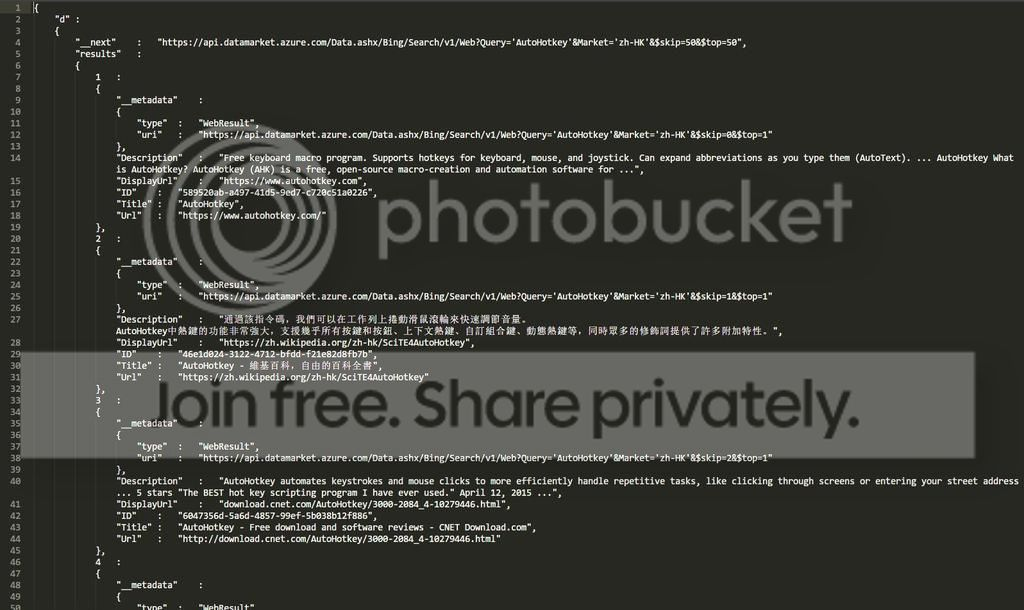Page 1 of 1

### ov() - Object view

Posted: 15 Aug 2016, 09:43

Code: Select all

``````ov(f,t:="",r:=""){
if !r
r:={&f:1}
else if r[&f]
Return "**"
else r[&f]:=1
for i,n in f
s.="`t" (IsObject(i)?ov(i,t "`t",r):(i+0?(i ""): "`"" i "`"")) "`t:`t" (IsObject(n)?ov(n,t "`t",r):(n+0?(n ""): "`"" n "`"")) ",`n" t
Return (t?("`n" t):"") "{`n" t rtrim(s,"`n`t,") "`t`n" t "}"
}``````